# Nelson Think Maths For The Australian Curriculum - Year 7

\$69.95
Buy 1 to 4: \$69.95 each
Buy 5 to 9: \$66.45 each
Buy 10 or more: \$62.95 each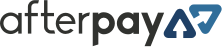Available on orders \$80 to \$1000Available on orders \$80 to \$1000

Nelson Think Maths for the Australian Curriculum 7 – 10 is a brand new series that has been developed to support teachers implementing the Australian Mathematics Curriculum for Years 7 – 10 students in Victoria.

Availability: Available Usually Dispatched In: 3 - 7 Business Days ISBN: 9780170214117 Publisher: Nelson Categories: Mathematics

Part of the series Think Maths For The Australian Curriculum.

Nelson Think Maths for the Australian Curriculum 7 – 10 is a brand new series that has been developed to support teachers implementing the Australian Mathematics Curriculum for Years 7 – 10 students in Victoria. Each student book gives access to the NelsonNet online student resources. NelsonNet and the NelsonNetBook (interactive eBook) are available to teachers and students who use the accompanying student book as a core educational resource in their classroom.

## Contents

1. Statistics

• 1.1 Data and surveys
• 1.2 Tabulating data
• 1.3 Graphing data
• 1.4 Analysing data
• Chapter summary
• Chapter review

2. Whole numbers

• 2.1 Using whole numbers
• 2.2 Order of operations
• 2.3 Index notation
• 2.4 Integers on the number line
• 2.5 Operating with integers
• 2.6 Special numbers
• Chapter summary
• Chapter review

3. Flat shapes

• 3.1 Points, lines and angles
• 3.2 Angle relationships
• 3.3 Naming flat shapes
• 3.4 Sketching and constructing flat shapes
• 3.5 Similarity, congruency and transformations
• Chapter summary
• Chapter review

4. Decimals

• 4.1 Naming and comparing decimals
• 4.2 Decimals, fractions and rounding
• 4.3 Adding and subtracting decimals
• 4.4 Multiplying and dividing decimals
• 4.5 Applying decimals
• Chapter summary
• Chapter review

5. Time

• 5.1 12-hour and 24-hour time
• 5.2 Using timelines
• 5.3 Converting units of time
• 5.4 Time calculations and timetables
• Chapter summary
• Chapter review

6. Expressions and equations

• 6.1 Patterns and symbols
• 6.2 Number rules
• 6.3 Algebraic expressions
• 6.4 Solving equations
• Chapter summary
• Chapter review

7. Fractions and ratios

• 7.1 Naming and comparing common fractions
• 7.2 Ordering fractions
• 7.3 Adding and subtracting fractions
• 7.4 Multiplying fractions
• 7.5 Dividing fractions
• 7.6 Rates and ratios
• Chapter summary
• Chapter review

8. Length and area

• 8.1 The metric system
• 8.2 Calculating perimeter
• 8.3 Area and its measurement
• 8.4 Calculating area
• Chapter summary
• Chapter review

9. Probability

• 9.1 Describing probability
• 9.2 Experimental probability
• 9.3 Theoretical probability
• Chapter summary
• Chapter review

10. Money and percentages

• 10.1 Money transactions
• 10.2 Percentages, fractions and decimals
• 10.3 Money and percentages
• Chapter summary
• Chapter review

11. Solid shapes and measures

• 11.1 Solids, nets and surface area
• 11.2 Naming and classifying solids
• 11.3 Drawing solids
• 11.4 Volume and capacity
• 11.5 Mass
• Chapter summary
• Chapter review

12. Number patterns

• 12.1 Graphs of number patterns
• 12.2 Number rules and functions
• 12.3 Functions and graphs
• Chapter summary
• Chapter review

Glossary

Appendix A

Scientific calculator skills

Appendix B

Scientific calculator exercises

Index

 ISBN 9780170214117 Publisher Nelson Product Type Student Books, Year Level Year 7,

#### Be The First To Review This Product!

Help other Teacher Superstore users shop smarter by writing reviews for products you have purchased.

### Others Also Bought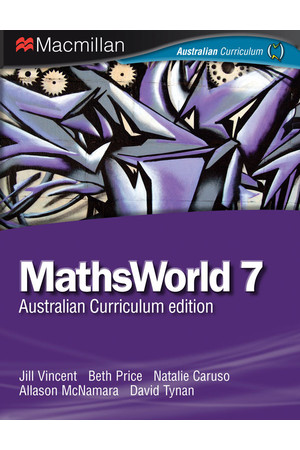### MathsWorld 7 - Print & eBook

\$82.50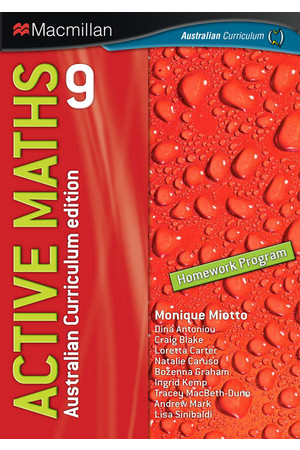### Active Maths 9 - Homework Program

\$24.50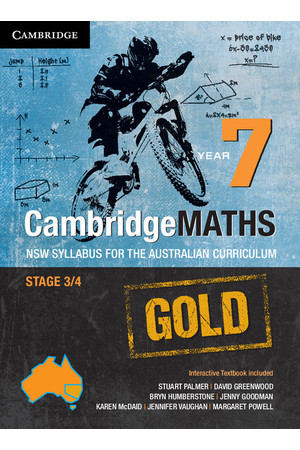### CambridgeMATHS GOLD - NSW Syllabus for the AC: Year 7 - Student Book + HOTmaths (Print & Digital)

\$74.95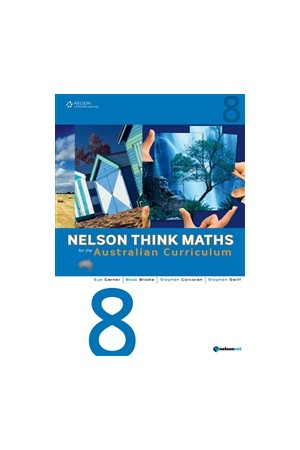### Nelson Think Maths For The Australian Curriculum - Year 8

\$69.95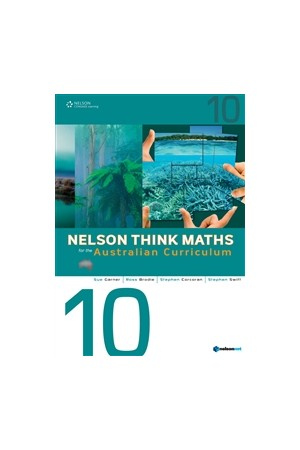### Nelson Think Maths For The Australian Curriculum - Year 10

\$69.95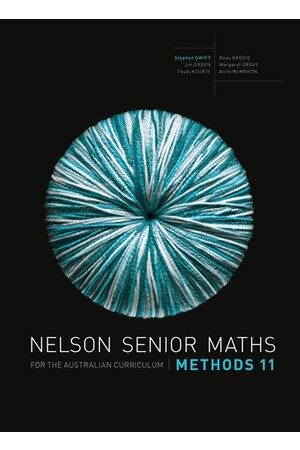### Nelson Senior Maths Methods for the Australian Curriculum - Year 11: Student Book

\$88.95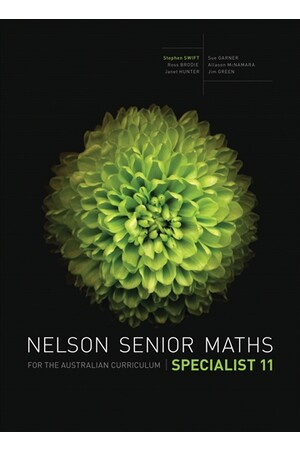### Nelson Senior Maths Specialist for the Australian Curriculum - Year 11: Student Book

\$88.95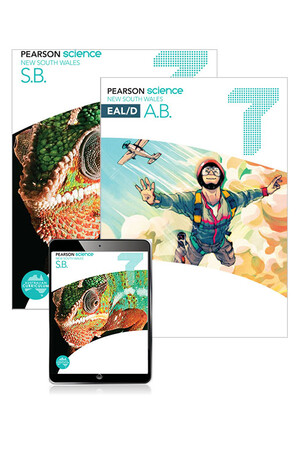\$65.95

### More From This Category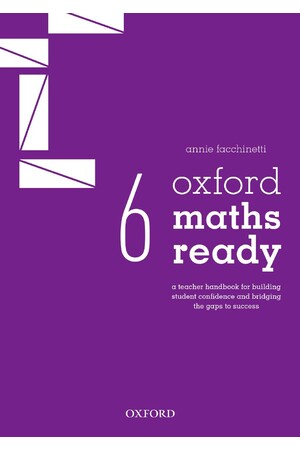### Oxford Maths Ready: Teacher Handbook - Year 6

\$49.99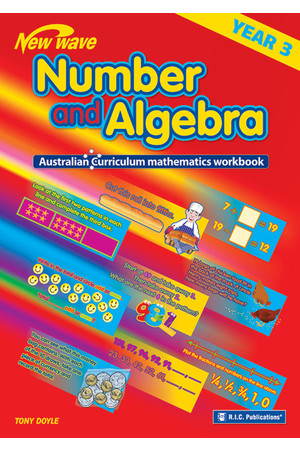### Australian Curriculum Mathematics - Number and Algebra Workbook: Year 3

\$7.95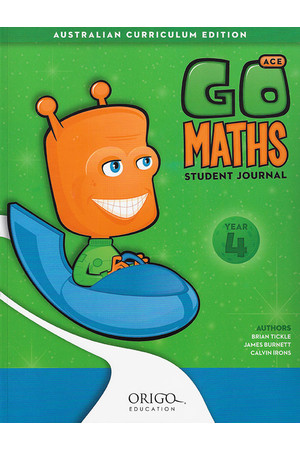### GO Maths ACE - Student Journal: Year 4

\$17.95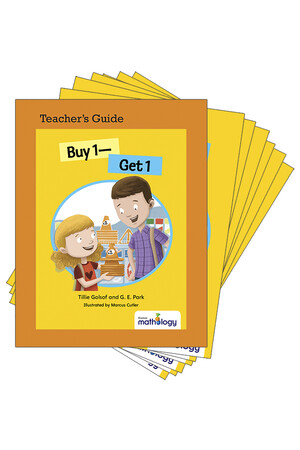### Mathology Little Books - Number: Buy 1 - Get 1 (6 Pack with Teacher's Guide)

\$61.95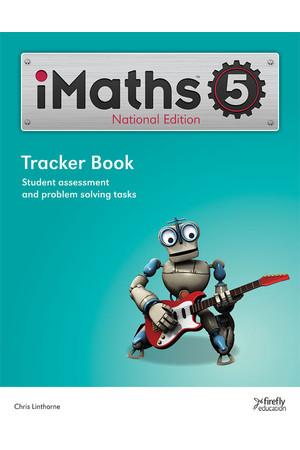### iMaths - Tracker Book: Year 5

\$8.95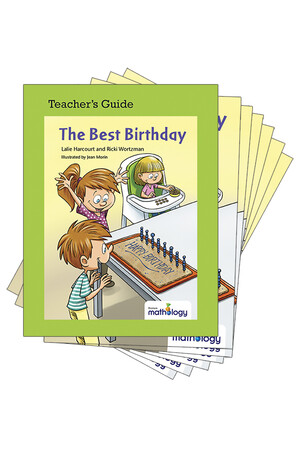### Mathology Little Books - Number: The Best Birthday (6 Pack with Teacher's Guide)

\$61.95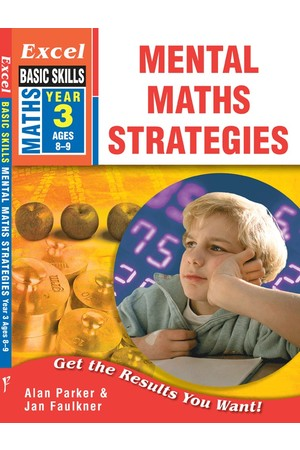### Excel Basic Skills - Mental Maths Strategies: Year 3

\$14.95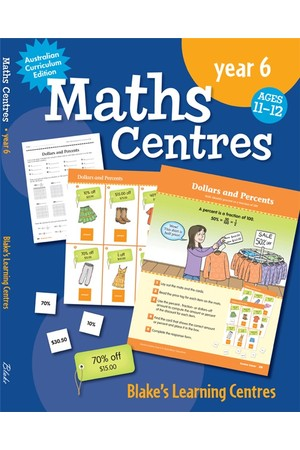\$49.95